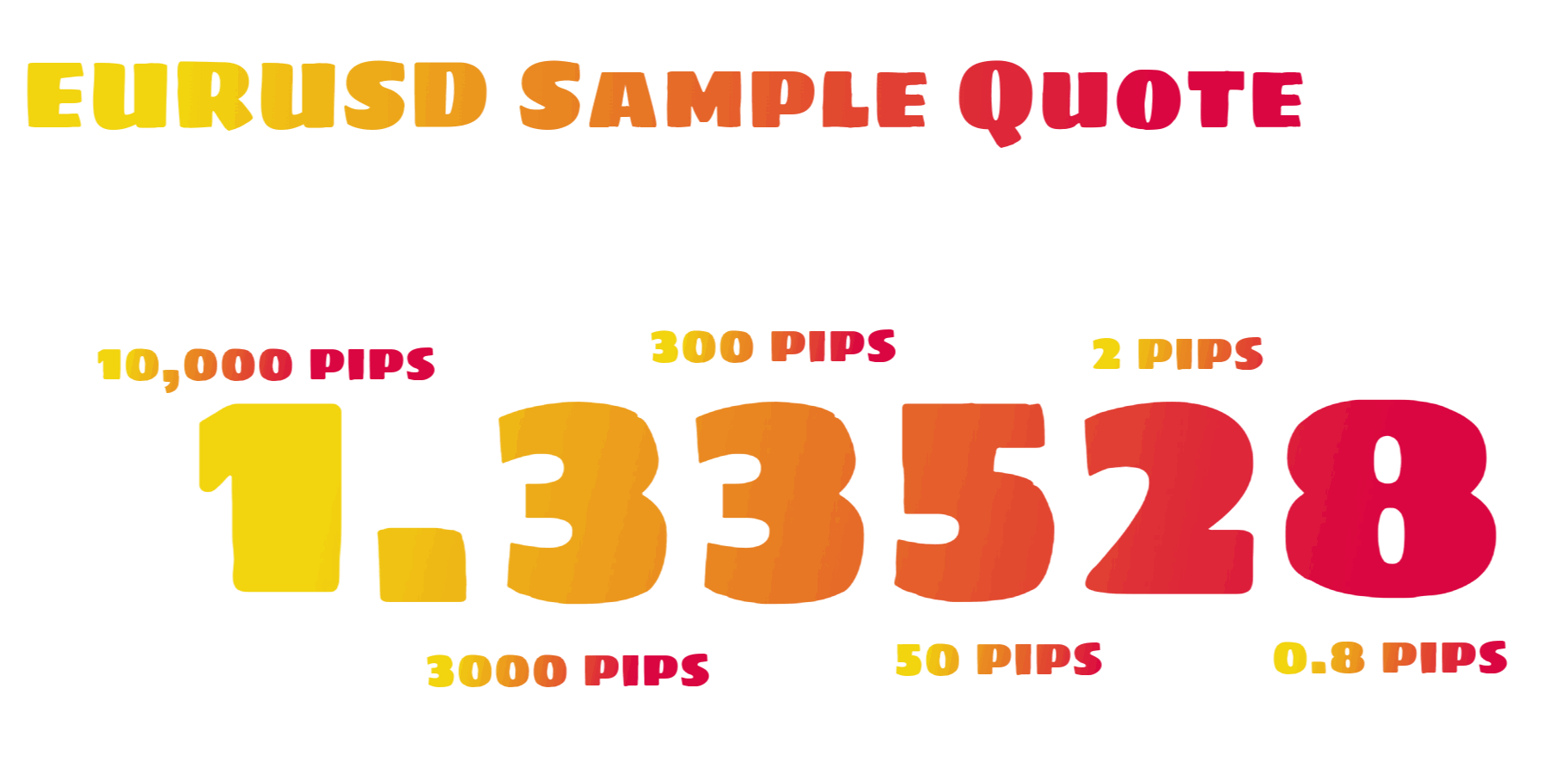A pip is the smallest number on a currency pair price movement or also known as points, pipettes.

For example, EURUSD exchange rates move from 1.12300 to 1.12325, this means the currency pair rises 25 pips.Another example, USDJPY price moves from 107.68 to 107.69. This means the currency pairs move 1 pip.

DOLLAR EARN PER PIP CALCULATION 💲

To calculate the value of dollar gained or loss per pip. Here is the formula that you can use,

Dollar (gained/loss) per standard lot = (0.0001/current price of the currency pair) x 100,000

Example calculation,

EURUSD assuming a current price of 1.3500

(0.0001/1.3500) x 100,000 = 7.41💲 per pip for a standard lot

So if you are trading with 5 Standard Lots, the value of dollar per pip will be 5 times that amount.

(0.0001/1.3500) x 10,000 = 0.74 💲 per pip for a mini lot

(0.0001/1.3500) x 1,000 = 0.07 💲 per pip for a micro lot

You don't have to go through all this calculation every time you trade since there is a lot of pip value calculators that exist online but still, you must know the idea.